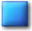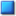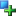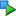##Image_Graph_Data_Common

Description

Base class for a data-element (e.g. "line" or "bar")

This class provides a basic implementation that is used and extended in all derived data elements. An instance of this class can be used as a "null output" (not actually drawing anything). This is usually *not* needed, however it might be useful in special cases.

Located in /Graph/Data/Common.php (line 64)

Direct descendents
Class DescriptionImage_Graph_Data_Line Line data-elementImage_Graph_Data_Bar Bar data-element
Method SummaryImage_Graph_Data_Common Image_Graph_Data_Common (object Image_Graph &\$graph, array \$data, [array \$attributes = array()])void drawGD (resource &\$img, [int \$drawWhat = IMAGE_GRAPH_DRAW_FILLANDBORDER])void setColor (mixed \$color)object data-marker-object &setDataMarker ([string \$representation = "line"], [array \$attributes = array()])object fill-object &setFill ([string \$type = "solid"], [array \$attributes = array()])void stackingDrawGD (array &\$dataElements, mixed &\$img)
MethodsConstructor Image_Graph_Data_Common (line 125)

Constructor

• access: public
Image_Graph_Data_Common Image_Graph_Data_Common (object Image_Graph &\$graph, array \$data, [array \$attributes = array()])
• object Image_Graph &\$graph: parent object
• array \$data: numerical data to be drawn
• array \$attributes: attributes like colordrawGD (line 325)

Draws diagram element

• access: public
void drawGD (resource &\$img, [int \$drawWhat = IMAGE_GRAPH_DRAW_FILLANDBORDER])
• resource &\$img: GD-resource to draw to
• int \$drawWhat: choose what to draw; use constants IMAGE_GRAPH_DRAW_FILLANDBORDER, IMAGE_GRAPH_DRAW_JUSTFILL or IMAGE_GRAPH_DRAW_JUSTBORDER

Redefined in descendants as:setColor (line 143)

Set color

void setColor (mixed \$color)
• mixed \$color: any color representation supported by Image_Graph_Color::color2RGB()setDataMarker (line 156)

Set a data marker to be used

• access: public
object data-marker-object &setDataMarker ([string \$representation = "line"], [array \$attributes = array()])
• string \$representation: data representation (e.g. "triangle")
• array \$attributes: attributes like colorsetFill (line 187)

Set a fill element to be used

• access: public
object fill-object &setFill ([string \$type = "solid"], [array \$attributes = array()])
• string \$type: type of fill (e.g. "solid")
• array \$attributes: attributes like colorstackingDrawGD (line 311)

Draw all diagram elements in this stacking-group

• static:
• access: public
void stackingDrawGD (array &\$dataElements, mixed &\$img)
• array &\$dataElements: references to dataElements (objects of this type)

Redefined in descendants as:

Documentation generated on Sat, 21 Feb 2004 23:28:01 +0100 by phpDocumentor 1.3.0RC2